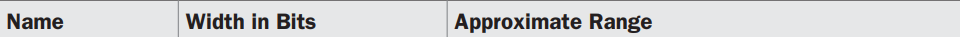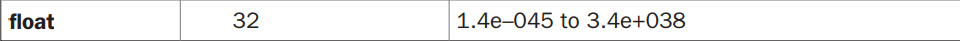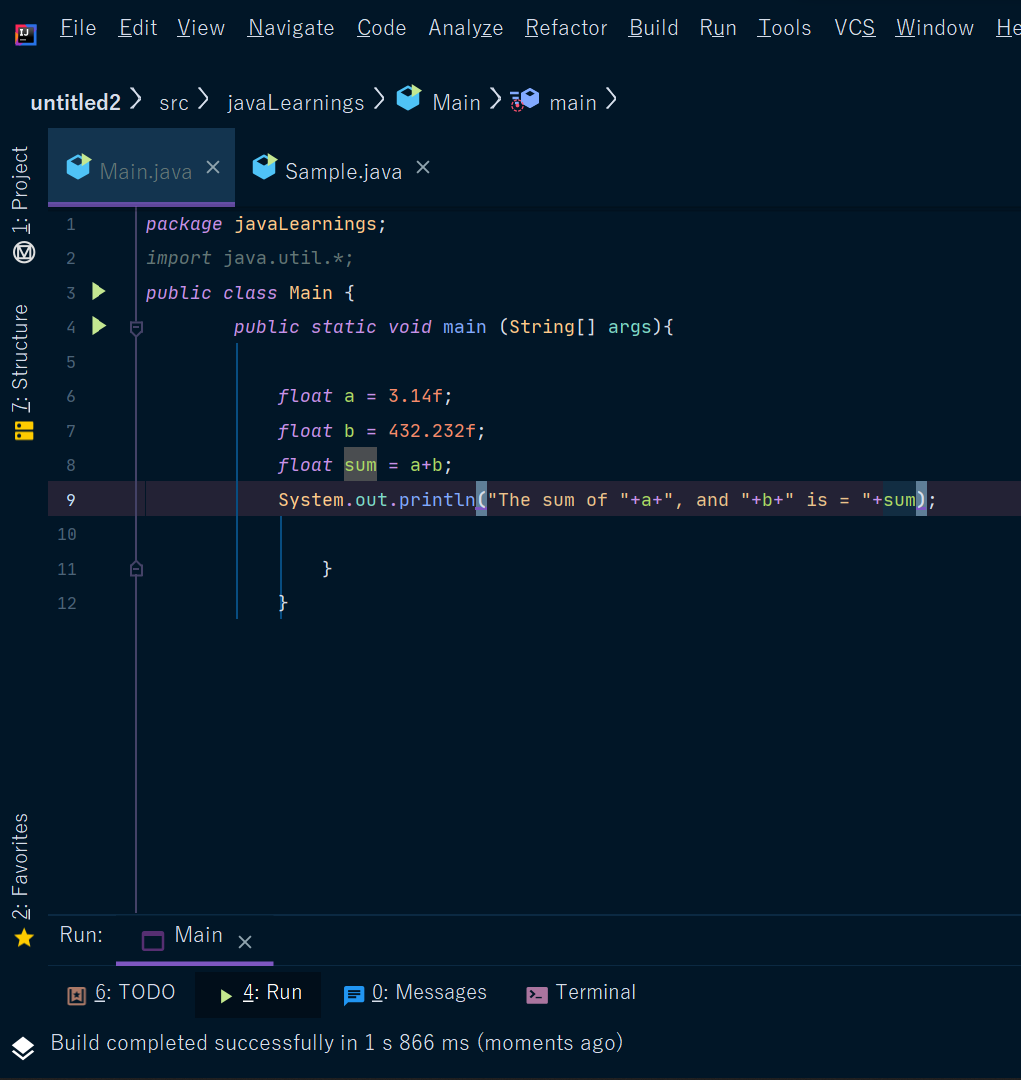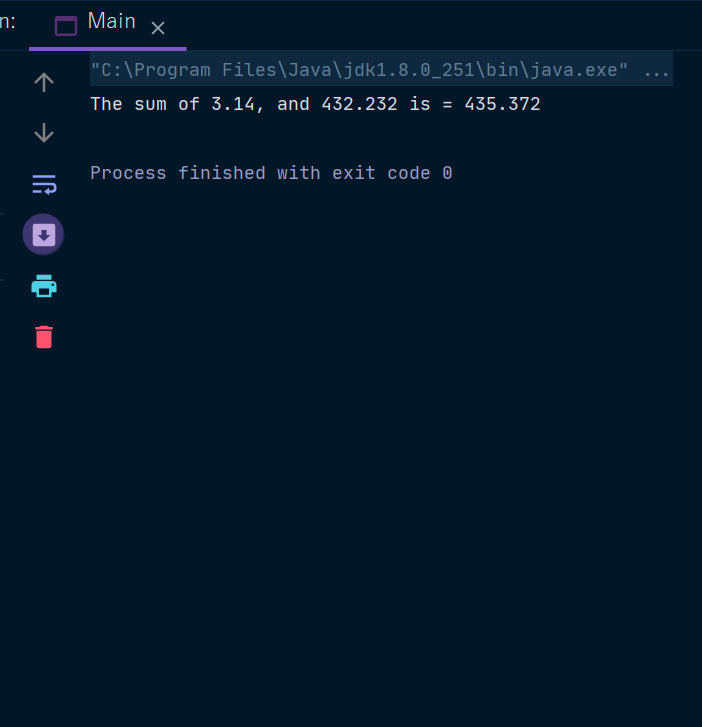Java Float Keyword

# Java Float Keyword

Float Keyword In Java
The type float specifies a single-precision value that uses 32 bits of storage.
Single precision is faster on some processors and takes half as much space as double-precision, but will become imprecise when the values are either very large or very small.
Variables of type float are useful when you need a fractional component, but don’t require a large degree of precision.
For example -  float can be useful when representing Rupees, and pesa.
Floating-point numbers represent decimal values with a fractional component. They can be expressed in either standard or scientific notation.
The Standard notation consists of a whole number component followed by a decimal point followed by a fractional component.
For example, 2.0, 3.14159, and 0.6667 represent valid standard-notation floating-point numbers.
The exponent is indicated by an E or e followed by a decimal number, which can be positive or negative. Examples include 6.022E23, 314159E–05, and 2e+100.
Floating-point literals in Java default to double precision. To specify a float literal, you must append an F or f to the constant.
The default double type consumes 64 bits of storage, while the less-accurate float type requires only 32 bits.
The range of float keyword is  -Now we will see an example -
Example -Output -